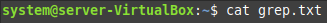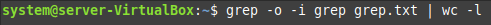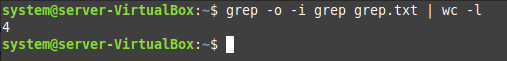Mint

# Use Grep to Count Total Number of Occurrences of Word in Text File in Linux Mint 21“Grep is a very useful Linux command that can perform a variety of different operations. One of the purposes for which this command can be used is to count the total occurrences of a specific word within a file. This guide will walk you through the complete procedure of counting the total number of occurrences of a word within a text file in Linux Mint 21.”

## Using the Grep Command to Count the Occurrences of a Word in a Text File in Linux Mint 21

If you want to find the total number of occurrences of any given word in a text file in Linux Mint 21 with the help of the grep command, then you should perform the following steps:

### Step # 1: View the Target File

First, we will show you the target file in which we will be counting the occurrences of a particular word. To display the contents of that file, we will execute the command shown below:

```\$ cat grep.txt
```The target text file is shown in the following image. From this file, we will be calculating the total occurrences of the word “grep” by using the grep command in Linux Mint 21.## Step # 2: Use Grep to Count the Total Number of Occurrences of a Particular Word of the Target File

Now, we will use the command shown below to count the number of occurrences of the specified word:

```\$ grep –o –i grep grep.txt | wc –l
```Here, the “-o” flag is used to print only the matching words, the “-i” flag is used to ignore the case of the following word, “grep” represents the word whose occurrences are to be calculated, “grep.txt” is the name of the file in which we need to perform this operation whereas “wc –l” is used to count the number of lines in which the match is found.

The total number of occurrences of our specified word from the given text file is shown in the following image:## Conclusion

This article was based on a very simple method for counting the total occurrences of a word within a text file in Linux Mint 21. By following this procedure, you will be able to find out the number of occurrences of any given word of your choice in a text file very easily.